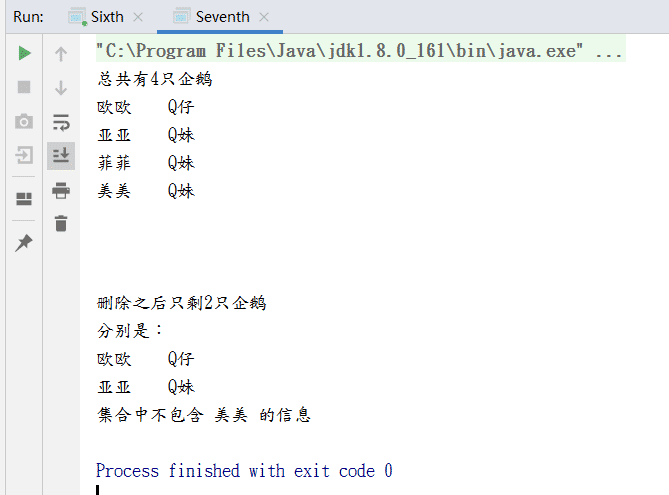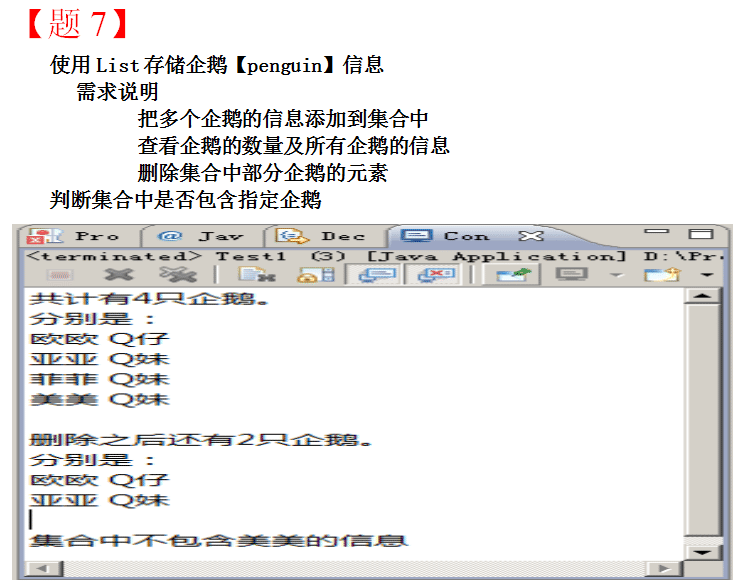# Java用list儲存，遍歷，查詢指定信息過程詳解

更新時間：2019年10月09日 11:53:31   作者：小龍_T無限我要評論```import java.util.ArrayList;
import java.util.List;

/**
* @auther:：9527
* @Description: 第七題
* @program: 多線程
* @create: 2019-08-09 23:39
*/
public class Seventh {
public static void main(String[] args) {
//初始化企鵝信息
Pengui p1 = new Pengui("歐歐", "Q仔");
Pengui p2 = new Pengui("亞亞", "Q妹");
Pengui p3 = new Pengui("菲菲", "Q妹");
Pengui p4 = new Pengui("美美", "Q妹");
//創建List集合并將企鵝信息添加進list中
List<Pengui> list = new ArrayList<>();
//查看企鵝數量
System.out.println("總共有" + list.size() + "只企鵝");
//查看所有企鵝的信息
for (Pengui pengui : list) {
System.out.println(pengui.getName() + "\t" + pengui.getGender());
}
//按題意刪除指定的企鵝 題目中只保留了歐歐和亞亞，所以要刪除的是菲菲和美美
for (int i = 0; i < list.size(); i++) {
if (list.get(i).getName().equals("菲菲")) {
list.remove(i);
}

if (list.get(i).getName().equals("美美")) {
list.remove(i);
}
}
System.out.println("\n\n");
System.out.println("刪除之后只剩" + list.size() + "只企鵝\n" +
"分別是：");
//查看剩下的企鵝
for (Pengui pengui : list) {
System.out.println(pengui.getName() + "\t" + pengui.getGender());
}

//判斷企鵝中是否包含指定企鵝,題目中指定的是美美
String index = "美美";
//設置中轉查詢temp  temp取值為一個數組下標中絕對不會出現的數字
int temp = -1;
//遍歷集合，如果集合中有這個元素，就給temp賦值，如果集合中沒有這個元素，則不會被賦值
for (int i = 0; i < list.size(); i++) {
if (list.get(i).getName().equals(index)){
temp = i;
}
}
//如果temp的值沒變，則表明沒有這個元素，如果temp的值變掉了，則表明有這個元素
if (temp == -1){
System.out.println("集合中不包含 "+index+" 的信息");
}else{
System.out.println("集合中包含 "+index+ " 的信息");
}
}
}```